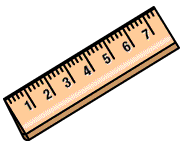BIG IDEAS:

(taken from “Big Ideas by Dr. Small”):
1. There is always value in estimating a measurement.
2. The length of an object is a one-dimensional attribute. Length can be the measurement of a single measure of an object or a combined linear measure, like perimeter.

STUDENT LEARNING GOALS:

GOAL: I can convert metric units between kilometres, metres, centimetres and millimetres.
GOAL: I can use a ruler to measure to nearest tenth of centimeter (i.e. mm).

CURRICULUM EXPECTATIONS:

• select and justify the most appropriate standard unit (i.e., millimetre, centimetre, decimetre, metre, kilometre) to measure length, height, width, and distance;
• solve problems requiring conversion from metres to centimetres and from kilometres to metres (Sample problem: Describe the multiplicative relationship between the number of centimetres and the number of metres that represent a length. Use this relationship to convert 5.1 m to centimetres.);